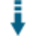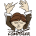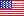# PHP Permutation and Combination: Compute permutations and combinations of valuesDownload .zip
 InfoExampleView files (2)Download .zip Reputation Support forum Blog Links
 Ratings Unique User Downloads Download Rankings Not enough user ratings Total: 126 All time: 9,371 This week: 102Version License PHP version Categories permutation-combine 1.0.0 Freeware 5 PHP 5, Statistics
Description

Author

This class compute permutations and combinations of given values.

It can take as parameters a given number of items or a number of items to pick.

The class can compute the total number of possible combinations or permutations of items either allowing repetitions or not.Performance Level
Name: Classes: Anthony Amolochitis `` 10 packages by Anthony AmolochitisUnited States 44 1138 166 in United States19116 in United States## Example

 ``` nItems ;         \$factorial = 1 ;         for( \$x = \$num ; \$x >= 1 ; \$x-- )         {             \$factorial = \$factorial * \$x ;         }         return \$factorial ;     }         /**      * Compute the R factorial      * @return int      */     protected function Generate_R_Factorial()     {         \$num = \$this->rPicked ;         \$factorial = 1 ;         for( \$x = \$num ; \$x >= 1 ; \$x-- )         {             \$factorial = \$factorial * \$x ;         }         return \$factorial ;     }         /**      * Compute the N factorial      * @return int      */     protected function Generate_N_Minus_R_Factorial()     {         \$num = \$this->nItems - \$this->rPicked ;         \$factorial = 1 ;         for( \$x = \$num ; \$x >= 1 ; \$x-- )         {             \$factorial = \$factorial * \$x ;         }         return \$factorial ;     }         /**      * Compute the (N + R - 1) factorial      * @return int      */     protected function Generate_N_Plus_R_MinusOne_Factorial()     {         \$num = ( \$this->nItems + \$this->rPicked - 1 ) ;         \$factorial = 1 ;         for( \$x = \$num ; \$x >= 1 ; \$x-- )         {             \$factorial = \$factorial * \$x ;         }         return \$factorial ;     }         /**      * Compute the (N + R - 1) factorial      * @return int      */     protected function Generate_N_Minus_One_Factorial()     {         \$num = ( \$this->nItems - 1 ) ;         \$factorial = 1 ;         for( \$x = \$num ; \$x >= 1 ; \$x-- )         {             \$factorial = \$factorial * \$x ;         }         return \$factorial ;     }         /**      * nPr      * Ordering matters.      * a and b are different that b and a.      * @return float      */     public function ComputePermutations()     {         \$nFactorial = \$this->Generate_N_Factorial();         \$n_Minus_r_Factorial = \$this->Generate_N_Minus_R_Factorial() ;                 return ( \$nFactorial / \$n_Minus_r_Factorial );     }         /**      * Ordering does not matter      * a and b are different that b and a.      * @return float      */     public function ComputePermutations_AllowRepitition()     {         return pow(\$this->nItems, \$this->rPicked);     }         /**      * nCr      * Order does not matter.      * a and b is the same as b and a      * @return float      */     public function ComputeCombinations()     {         \$nFactorial = \$this->Generate_N_Factorial();         \$rFactorial = \$this->Generate_R_Factorial() ;         \$n_Minus_r_Factorial = \$this->Generate_N_Minus_R_Factorial() ;                 return ( \$nFactorial / (\$n_Minus_r_Factorial * \$rFactorial) );     }             public function ComputeCombination_RepititionAllowed()     {         \$numerator = \$this->Generate_N_Plus_R_MinusOne_Factorial();         \$rFactorial = \$this->Generate_R_Factorial() ;         \$nMinusOneFactorial = \$this->Generate_N_Minus_One_Factorial();                 return ( \$numerator / (\$rFactorial * \$nMinusOneFactorial) );     }     } \$t = new PermutationCombination(); \$t->nItems = 5 ; \$t->rPicked = 5 ; echo 'Permutations: ' ; echo \$t->ComputePermutations() ; echo '
'; echo 'Permutations Allow Repitition: ' ; echo \$t->ComputePermutations_AllowRepitition() ; echo '
'; echo 'Combinations: ' ; echo \$t->ComputeCombinations() ; echo '
'; echo 'Combinations with Repitition: ' ; echo \$t->ComputeCombination_RepititionAllowed() ; ?> ```Files
File Role DescriptionExampleUsage.php Example Example Usage of the classPermutationCombination.php Class Compute Combinations and Permutations

 All time: 9,371 This week: 102About usAdvertise on this siteSite mapNewsletterStatisticsSite tipsPrivacy policyContact
For more information send a message to `info at phpclasses dot org`.